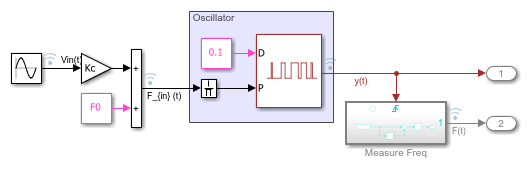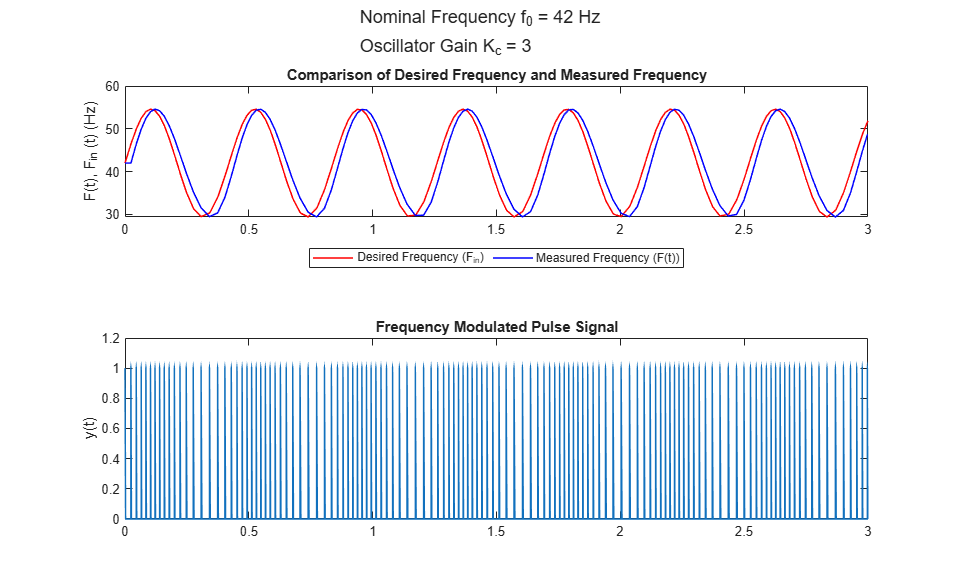# Voltage Controlled Oscillator

This example shows you how to model an ideal voltage controlled oscillator using the Variable Pulse Generator block to create the frequency oscillations.

A voltage controlled oscillator uses an input tuning voltage to produce waveforms of varying frequency. Over a small range of voltages, the relationship between the input voltage ()and the output oscillation frequency () is proportional and can be expressed as

``

where

•is the oscillator sensitivity in Hz/V

•is the quiescent frequency, or nominal frequency of the oscillator atIn the included `vco_using_vpg` model, the desired oscillation frequency signal `F_{in}(t)` is generated using the formula shown in equation (1). In this model, the tuning voltageis a sinusoidal waveform.